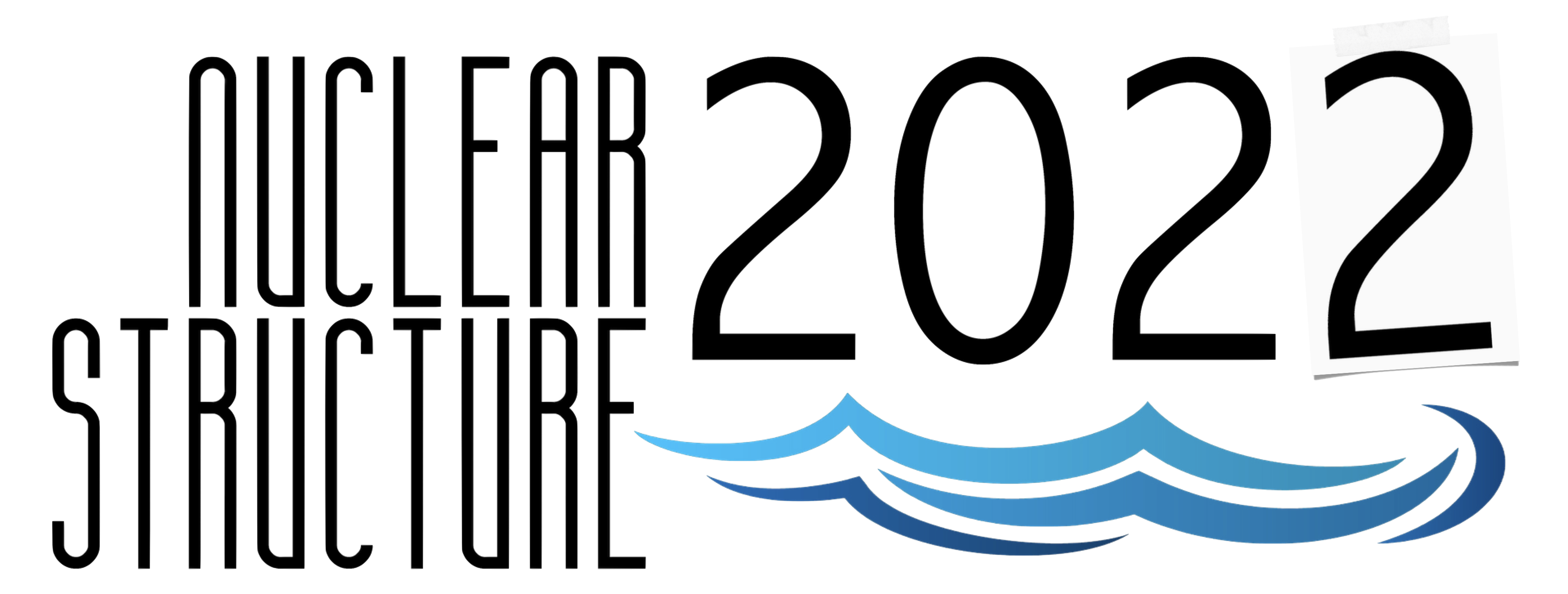#Nuclear Structure 2022

Jun 13 – 17, 2022
Berkeley, CA
US/Pacific timezone

## Study of intruder states towards $^{78}$Ni with lifetime measurements following $^{82}$Se(d,p)$^{83}$Se

Not scheduled
1m
Berkeley, CA

#### Berkeley, CA

Lawrence Berkeley National Laboratory
Oral Poster Presentations

### Speaker

Julgen Pellumaj (INFN-LNL)

### Description

Quadrupole interaction between protons and neutrons drives the nucleus into deformed configurations at low excitation energies. Around the N=50 shell gap, intruder states with spins 1/2$^{+}$ and 5/2$^{+}$, originating from the s$_{1/2}$ and d$_{5/2}$ orbitals were first observed in $^{83}$Se and, later on, in the other N=49 isotones $^{87}$Kr, $^{81}$Ge and $^{79}$Zn. In $^{83}$Se these intruder states reach energies of around 500 keV, the lowest among the other N=49 isotones. The $^{83}$Se nucleus is at the mid of the proton fp-shell and it should have the maximum of quadrupole correlations which makes it a good candidate to understand the collectivity of the particle-hole intruder states in this region, lowered in energy by large quadrupole correlations. Indeed, large-scale shell model calculations predict a quenching of the energy of the intruder states in $^{83}$Se, at variance with the experimental data .
Lifetime measurements of the intruder states of $^{83}$Se would give an indication about their wave function and would allow estimating the degree of the N=50 core breaking in the ground state of Se isotopes. Moreover, such measurements could shed light on the behavior of the N=50 shell gap towards $^{78}$Ni, a double-magic nucleus in which intruder configurations competing in energy with the spherical ones have also been found .

We will report on the results obtained from a recent experiment performed in Laboratori Nazionali di Legnaro, where lifetimes of the intruder-state band were measured using Recoil Distance Doppler-Shift Method and Doppler-Shift Attenuation Method. A beam of $^{82}$Se, with intensity 0.02 pnA, accelerated at 270 MeV by the ALPI-TANDEM accelerator at LNL-INFN, impinged into a deuterated polyethylene (C$_2$D$_4$) target which was evaporated on a 6 mg/cm$^{2}$ thick gold layer. The GALILEO $\gamma$-array was coupled to the SPIDER silicon-array, allowing to obtain the needed channel selectivity through coincidence measurements between γ rays and protons coming from the (d,p) transfer reaction. The results on lifetimes will be discussed in the framework of large-scale shell-model calculations and mean-field approaches, pointing out the role of the collectivity of low-lying intruder configurations.
 C. Wraith et al., Nature, 569, 53-58 (2019).
 R. Taniuchi et al., Phys. Lett. B. 771, 385-391 (2017).

### Primary authors

Julgen Pellumaj (INFN-LNL) Andrea Gottardo (INFN LNL)

### Presentation materials

There are no materials yet.